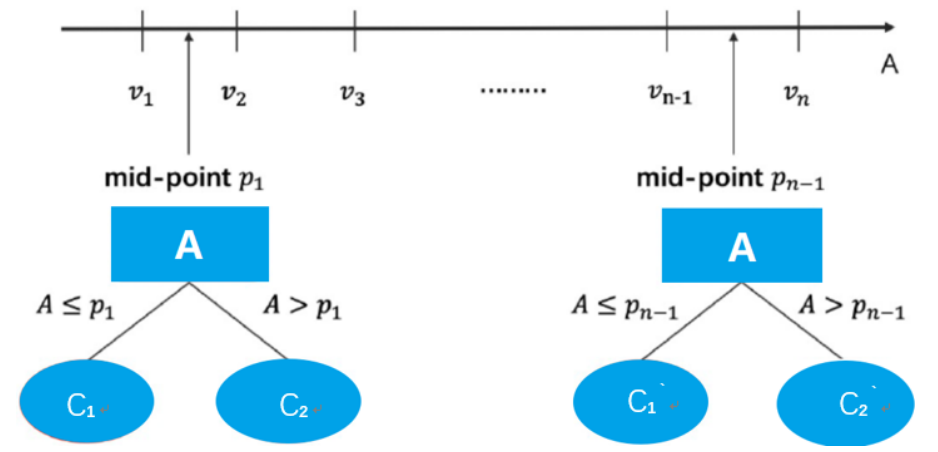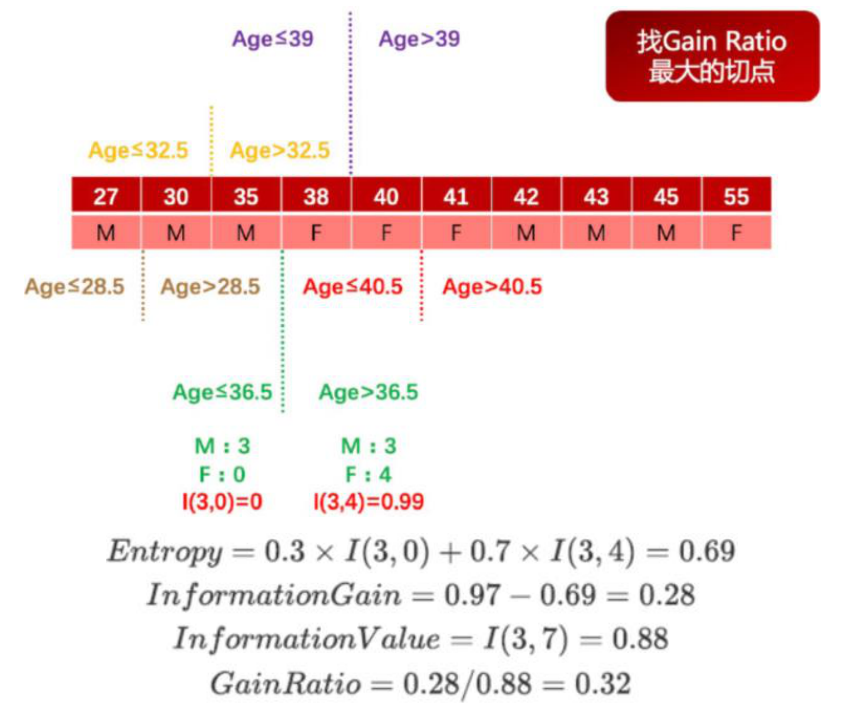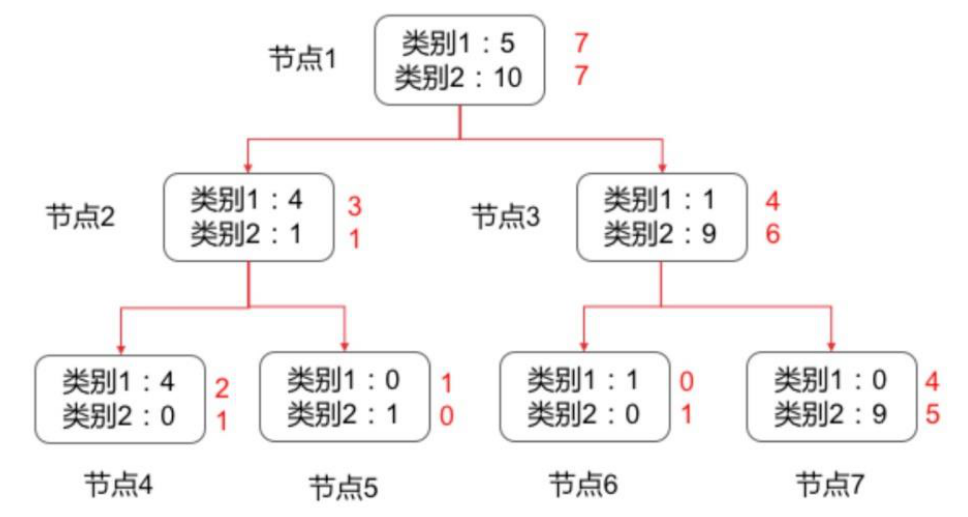# 前言

### 目录

• 前言
• 1 C4.5 算法
• 1.1 修改局部最优化条件
• 1.2 连续变量处理手段
• 2 决策树的拟合度优化
• 2.1 决策树剪枝
• 2.2 CART 算法
• 2.2.1 分裂准则
• 2.2.2 二叉分裂的优点
• 2.2.3 利用测试集进行剪枝
• 2.2.3 测试集和验证集
• 结束语

# 1 C4.5 算法

C4.5 算法与 ID3 算法相似，C4.5 算法对 ID3 算法进行了改进，C4.5 在生成的过程中，用信息增益比准则来选择特征。

## 1.1 修改局部最优化条件

• 以信息增益作为划分训练数据集的特征，存在偏向于选择取值较多的特征的问题
• 使用信息增益比（information gain ratio）可以对这一问题进行校正。

IV 值会随着叶节点上样本量的变小而逐渐变大，也就是说一个特征属性中如果标签分类太多，每个叶子上的 IV 值就会非常大。我们可利用 Gain_ratio 代替 Gain 重新计算数据集中第 0 列的 Gain_ratio ，由于根据 accompany 字段切分后，2 个分支分别有 3 个和 2 个的样例数据，因此其 IV 指标计算过程如下： I V ( ′ a c c o m p a n y ′ ) = − 3 5 l o g 2 3 5 − 2 5 l o g 2 2 5 = 0.971 G a i n _ r a t i o ( ′ a c c o m p a n y ′ ) = G a i n ( ′ a c c o m p a n y ′ ) I V ( ′ a c c o m p a n y ′ ) = 0.42 0.971 = 0.432 IV('accompany')=-\frac{3}{5}log_2 \frac{3}{5}-\frac{2}{5}log_2 \frac{2}{5}=0.971\\Gain\_ratio('accompany')=\frac{Gain('accompany')}{IV('accompany')}=\frac{0.42}{0.971}=0.432 IV(accompany)=53log25352log252=0.971Gain_ratio(accompany)=IV(accompany)Gain(accompany)=0.9710.42=0.432然后可进一步计算其他各字段的 Gain_ratio ，并选取 Gain_ratio 最大的字段进行切分。

## 1.2 连续变量处理手段import numpy as np
import pandas as pd

# 定义信息熵
def calEnt(dataSet):
n = dataSet.shape                                     # 数据集总行数
iset = dataSet.iloc[:,-1].value_counts()                 # 统计标签的所有类别
p = iset/n                                               # 统计每一类标签所占比
ent = (-p*np.log2(p)).sum()                              # 计算信息熵
return ent

# 选择最优的列进行切分
def bestSplit2(dataSet):
baseEnt = calEnt(dataSet)                                # 计算原始熵
bestGainRatio = 0                                       # 初始化信息增益
axis = -1                                                # 初始化最佳切分列，标签列

for i in range(dataSet.shape-1):                      # 对特征的每一列进行循环
levels= dataSet.iloc[:,i].value_counts().index       # 提取出当前列的所有取值
ents = 0                                             # 初始化子节点的信息熵
IV = 0                                               # 初始化子节点的属性分裂信息度量

for j in levels:                                     # 对当前列的每一个取值进行循环
childSet = dataSet[dataSet.iloc[:,i]==j]         # 某一个子节点的dataframe
ent = calEnt(childSet)                           # 计算某一个子节点的信息熵
p = childSet.shape/dataSet.shape           # 计算当前取值的概率
ents += p*ent                                    # 计算当前列的信息熵
IV += -(p*np.log(p))                             # 计算当前列的分裂信息度量
print('第{}列的信息熵为{}'.format(i,ents))

infoGain = baseEnt-ents                              # 计算当前列的信息增益
print('第{}列的信息增益为{}'.format(i,infoGain))

gainRatio = infoGain / IV                           # 计算当前列的信息增益率
print('第{}列的信息增益率为{}\n'.format(i,gainRatio))

if (gainRatio > bestGainRatio):
bestGainRatio = gainRatio                      # 选择最大信息增益率
axis = i                                         # 最大信息增益率所在列的索引
print("第{}列为最优切分列\n".format(axis))
return axis

#为决策树进一步划分去做准备
def mySplit(dataSet,axis,value):
col = dataSet.columns[axis]
redataSet = dataSet.loc[dataSet[col]==value,:].drop(col,axis=1)
return redataSet

"""

dataSet：原始数据集(最右一列是标签)
return：myTree：字典形式的树
"""
def createTree2(dataSet):
featlist = list(dataSet.columns)                        # 提取出数据集所有的列
classlist = dataSet.iloc[:,-1].value_counts()           # 获取最后一列类标签

# 判断最多标签数目是否等于数据集行数，或者数据集是否只有一列
if classlist==dataSet.shape or dataSet.shape == 1:
return classlist.index                           # 如果是，返回类标签

axis = bestSplit2(dataSet)                              # 确定出当前最佳切分列的索引
bestfeat = featlist[axis]                               # 获取该索引对应的特征
myTree = {bestfeat:{}}                                  # 采用字典嵌套的方式存储树信息
del featlist[axis]                                      # 删除当前特征
valuelist = set(dataSet.iloc[:,axis])                   # 提取最佳切分列所有属性值

for value in valuelist:                                 # 对每一个属性值递归建树
myTree[bestfeat][value] = createTree(mySplit(dataSet,axis,value))

return myTree

row_data = {'是否陪伴' :[0,0,0,1,1],
'是否玩游戏':[1,1,0,1,1],
'渣男' :['是','是','不是','不是','不是']}
dataSet = pd.DataFrame(row_data)

createTree2(dataSet)


{‘是否陪伴’: {0: {‘是否玩游戏’: {0: ‘不是’, 1: ‘是’}}, 1: ‘不是’}}

# 2 决策树的拟合度优化

## 2.1 决策树剪枝

• 预剪枝：在决策树生成的过程中，对每个节点在划分前先进行估计，如果当前的节点划分不能带来决策树泛化性能（预测性能）的提升，则停止划分并且将当前节点标记为叶节点。
• 后剪枝：先训练生成一颗完整的树，自底向上对非叶节点进行考察，如果将该节点对应的子树替换为叶节点能带来决策树泛化能力的提升，则将该子树替换为叶节点。
\预剪枝后剪枝

## 2.2 CART 算法

CART：分类回归树（Classification and Regression Tree）

• 分裂过程是一个二叉递归划分过程
• CART 预测变量 x 的类型既可以是连续型变量也可以是分类型变量
• 数据应以其原始形式处理，不需要离散化
• 用于数值型预测时，并没有使用回归，而是基于到达叶节点的案例的平均值做出预测

### 2.2.1 分裂准则

• 对于连续变量：条件是属性小于等于最优分裂点
• 对于分类变量：条件是属性属于若干类

### 2.2.2 二叉分裂的优点

• 分类树：Gini 准则。与之前的信息增益很类似，Gini 系数度量一个节点的不纯度。
• 回归树：一种常见的分割标准是标准偏差减少（Standard Deviation Reduction, SDR），类似于最小均方误差 LS（least squares，预测错误的平方和）准则。

### 2.2.3 利用测试集进行剪枝• 判断每个叶节点在验证集上的错误率：
• 节点 4 的错误率 e ( 4 ) = 1 3 e(4)=\frac{1}{3} e(4)=31
• 节点 5 的错误率 e ( 5 ) = 1 e(5)=1 e(5)=1
• 节点 6 的错误率 e ( 6 ) = 1 e(6)=1 e(6)=1
• 节点 7 的错误率为 e ( 7 ) = 4 9 e(7)=\frac{4}{9} e(7)=94

1. 删除以此结点为根的子树
2. 使其成为叶子结点
3. 赋予该结点关联的训练数据的最常见分类
4. 当修剪后的树对于验证集合的性能不会比原来的树差时，才真正删除该结点（这就是为什么拿父节点错误率和子结点错误率权重和比较的原因）

REP是从底向上进行处理，直到进一步修剪有害为止(再剪枝会减低验证集合的精度)。

REP是最简单的后剪枝方法之一，不过由于使用独立的测试集，原始决策树相比，修改后的决策树可能偏向于过度修剪

# 结束语

💚 开始学习机器学习时你必须要了解的模型有哪些？机器学习系列之决策树基础篇
❤️ 以❤️简单易懂❤️的语言带你搞懂有监督学习算法【附Python代码详解】机器学习系列之KNN篇
💜 开始学习机器学习之前你必须要了解的知识有哪些？机器学习系列入门篇

🖤 我和关注我的前1000个粉丝“合影”啦！收集前1000个粉丝进行了一系列数据分析，收获满满
❤️ 分享一个超nice的数据分析实战案例 ⭐ “手把手”教学，收藏等于学会
💙 数据分析必须掌握的RFM模型是什么？一文搞懂如何利用RFM对用户进行分类【附实战讲解】
💚 MySQL必须掌握的技能有哪些？超细长文带你掌握MySQL【建议收藏】
💜 Hive必须了解的技能有哪些？万字博客带你掌握Hive❤️【建议收藏】
🧡 一文带你了解Hive【详细介绍】Hive与传统数据库有什么区别？

👨‍👩‍👦‍👦 机器学习：分享机器学习理论基础和常用模型讲解
👨‍👩‍👦‍👦 数据分析：分享数据分析实战项目和常用技能整理

CSDN@报告，今天也有好好学习

<< 上一篇 下一篇 >>

### 评论（0） 赞助本站◎欢迎参与讨论，请在这里发表您的看法、交流您的观点。